# Potentiometers

## DC Electric Circuits

• #### Question 1

 Don’t just sit there! Build something!!

Learning to mathematically analyze circuits requires much study and practice. Typically, students practice by working through lots of sample problems and checking their answers against those provided by the textbook or the instructor. While this is good, there is a much better way.

You will learn much more by actually building and analyzing real circuits, letting your test equipment provide the “answers” instead of a book or another person. For successful circuit-building exercises, follow these steps:

1. Carefully measure and record all component values prior to circuit construction.
2. Draw the schematic diagram for the circuit to be analyzed.
3. Carefully build this circuit on a breadboard or other convenient medium.
4. Check the accuracy of the circuit’s construction, following each wire to each connection point, and verifying these elements one-by-one on the diagram.
5. Mathematically analyze the circuit, solving for all values of voltage, current, etc.
6. Carefully measure those quantities, to verify the accuracy of your analysis.
7. If there are any substantial errors (greater than a few percent), carefully check your circuit’s construction against the diagram, then carefully re-calculate the values and re-measure.

Avoid very high and very low resistor values, to avoid measurement errors caused by meter “loading”. I recommend resistors between 1 kΩ and 100 kΩ, unless, of course, the purpose of the circuit is to illustrate the effects of meter loading!

One way you can save time and reduce the possibility of error is to begin with a very simple circuit and incrementally add components to increase its complexity after each analysis, rather than building a whole new circuit for each practice problem. Another time-saving technique is to re-use the same components in a variety of different circuit configurations. This way, you won’t have to measure any component’s value more than once.

• #### Question 2

A very common misconception students have about potentiometers is the relationship between resistance and direction of wiper motion. For instance, it is common to hear a student say something like this, “Turning the potentiometer so the wiper moves up will increase the resistance of the potentiometer.”Explain why it does not really make sense to say something like this.

• #### Question 3

Potentiometers are manufactured in two different “tapers”: linear and audio. Linear taper potentiometers provide a direct, linear relationship between wiper position and resistance division, so that equal changes in wiper position result in equal changes in resistance. Audio taper potentiometers provide a non-linear (logarithmic, to be exact) relationship between wiper position and resistance division, so that the same amount of wiper motion at one end of its range gives a much greater change in resistance than at the other end of its range.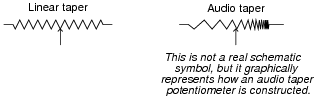Suppose you have a potentiometer, but do not know whether it has a linear or audio taper. How could you determine this, using a meter?

• #### Question 4

Suppose a length of resistive material (such as nichrome wire) had three points of electrical contact: one at each end (points 1 and 3), plus a movable metal “wiper” making contact at some point between the two ends (point 2):Describe what happens to the amount of electrical resistance between the following points, as the wiper is moved toward the left end of the resistive element (toward point 1)? State your answers in terms of “increase,” “decrease,” or “remains the same,” and explain why each answer is so.Between points 1 and 2, resistance . . .
Between points 2 and 3, resistance . . .
Between points 1 and 3, resistance . . .
• #### Question 5

A technician decides to use a potentiometer as a speed control for an electric motor. The potentiometer has a resistance rating of 10 Ω and a power rating of 25 watts:When operating the motor at a voltage of 16 volts and a current of 2 amps, though, smoke begins to pour out of the potentiometer, indicating that its power rating is being exceeded.

The technician is perplexed! According to his calculations, the potentiometer should be dissipating less than 25 watts of power. Why, then, is a potentiometer rated for 25 watts burning up under this condition?

• #### Question 6

When potentiometers are used as variable resistors (rheostats), the unused terminal is often connected to the wiper terminal: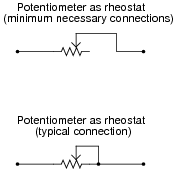Explain what advantage is gained by connecting the wiper to the unused terminal. Why is the lower potentiometer connection scheme more commonly used than the upper?

• #### Question 7

Draw a schematic diagram showing a potentiometer being used as a simple variable resistor for varying current to a light bulb. Also, designate which way the “wiper” should be moved to make the bulb glow brighter.

• #### Question 8

What is the difference between the following potentiometers, as indicated by their respective symbols?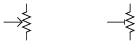• #### Question 9

What would you expect the voltmeter in the following circuit to do when the potentiometer wiper is moved to the right?What would you expect the voltmeter to register if the wiper were set precisely at the 50% (half-way) position?

• #### Question 10

Connect the necessary wires in this illustration to make the potentiometer act as a variable voltage divider, providing a variable voltage to the voltmeter: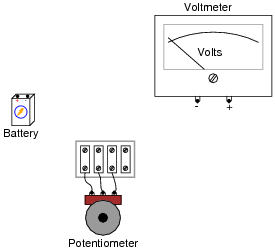• #### Question 11

With a trough of water and three pieces of wire, you could make a liquid potentiometer: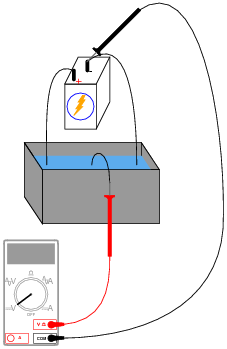Which way would you have to move the middle wire (the one touching the voltmeter’s red test lead) in order to increase the voltmeter’s reading?

• #### Question 12

Which way would you have to move the potentiometer wiper, to the left or to the right, in order to increase current through resistor R1?• #### Question 13

Which way would you have to twist the potentiometer shaft in order to increase current through resistor R1, clockwise or counter-clockwise? Explain your answer.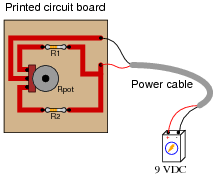• #### Question 14

This current divider circuit has a problem. The voltage between test points TP2 and TP1 varies with the potentiometer position, but the voltage between test points TP3 and TP1 remains at 0 volts no matter where the potentiometer is set: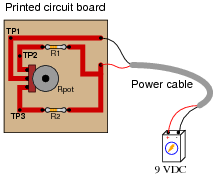Identify the most likely fault which would account for these measurements.

• #### Question 15

A radio technician is troubleshooting a problem in a simple AM receiver, using a voltage-measuring device called an oscilloscope. An oscilloscope is nothing more than a graphical voltmeter, indicating the “wave shape” of voltages that change rapidly over time. The problem with this radio is that no sound at all is heard in the headphones.

When checking for a voltage signal between points A and B in the circuit, a strong signal is obtained:However, when checking between points C and B in the circuit, no signal is measured: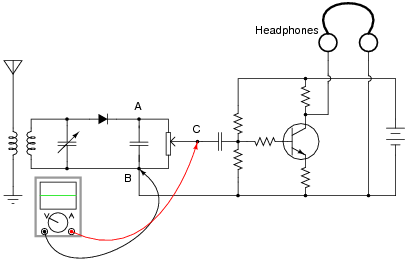What do these voltage measurements indicate about the nature of the problem in the receiver circuit? Is there a general troubleshooting rule that may be drawn from this example? If so, what is it?

If possible, identify the precise nature of the failure.

• #### Question 16

Calculate the voltmeter voltage in each of these circuits, assuming the wiper position is 25% up from the bottom: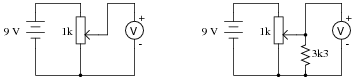• #### Question 17

Suppose we were building a circuit that required an adjustable resistance with a range of 1500 Ω to 4500 Ω. The only potentiometer we have on hand is a 10 kΩ unit. Of course, we could simply connect the potentiometer as-is and have an adjustable range of 0 Ω to 10,000 Ω, but that would be too “coarse” of an adjustment for our application.

Explain how we could connect other resistors to this 10 kΩ potentiometer in order to achieve the desired adjustable resistance range.

• #### Question 18

Find one or two real potentiometers and bring them with you to class for discussion. Identify as much information as you can about your potentiometers prior to discussion:

Resistance (ideal)
Resistance (actual)
Taper (linear or audio)
Number of turns
Power rating
Type (carbon composition, wire-wound, etc.)